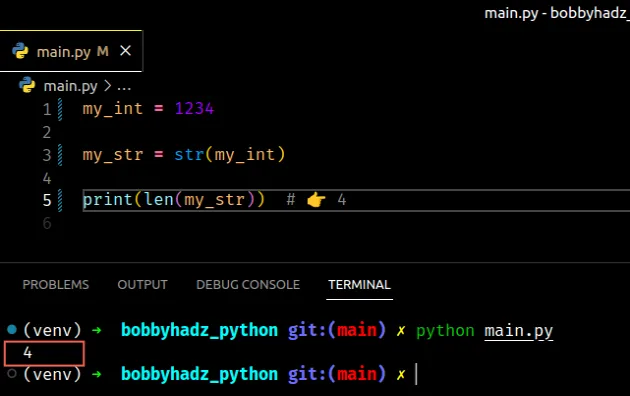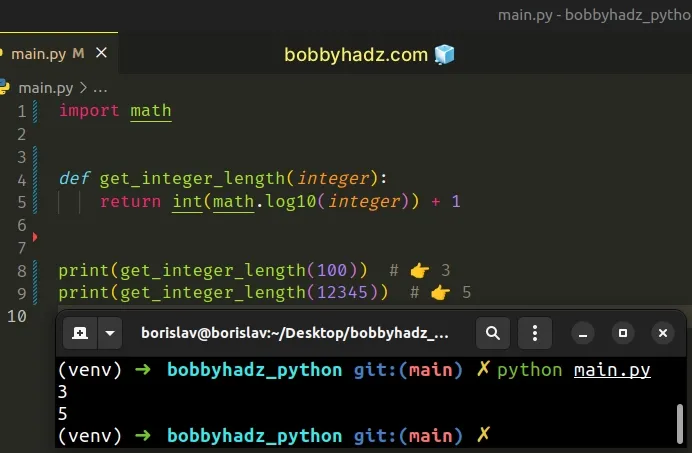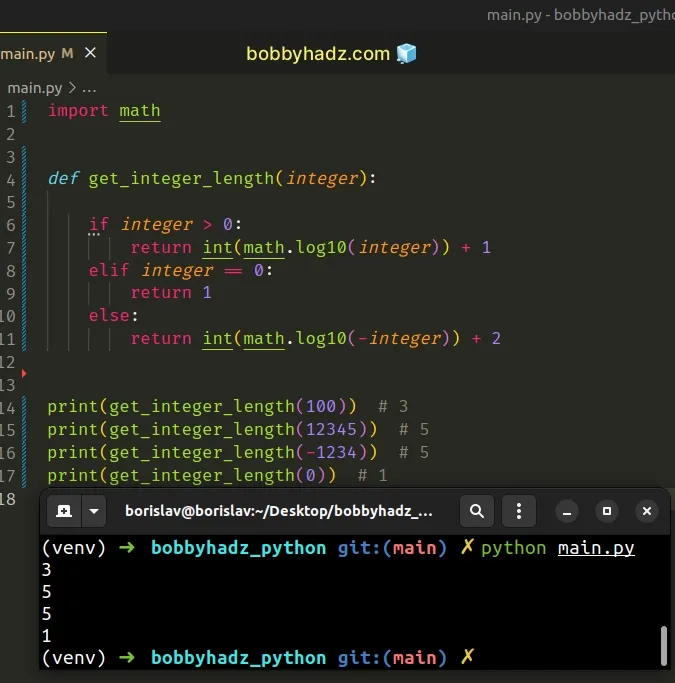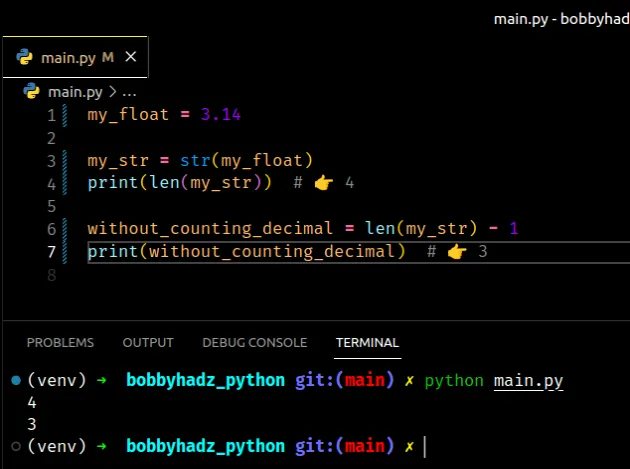# Get the length of an Integer or a Float in PythonLast updated: Feb 18, 2023
5 min## #Get the length of an Integer in Python

To get the length of an integer in Python:

1. Use the `str()` class to convert the integer to a string.
2. Pass the string to the `len()` function, e.g. `len(my_str)`.
3. The `len()` function will return the length of the string.
main.py
```Copied!```my_int = 1234

my_str = str(my_int)

print(len(my_str))  # 👉️ 4
``````The len() function returns the length (the number of items) of an object.

The argument the function takes may be a sequence (a string, tuple, list, range or bytes) or a collection (a dictionary, set, or frozen set).

This is why we had to convert the integer to a string - we can't pass an integer to the `len()` function as integers are not a sequence or a collection.

## #Handling possibly negative numbers

If you need to handle a scenario where the number is negative, subtract `1` from the result.

main.py
```Copied!```my_int = -1234

if my_int < 0:
result = len(str(my_int)) - 1
else:
result = len(str(my_int))

print(result)  # 👉️ 4
``````

We check if the integer is less than `0`, and if it is, we subtract `1` from its length to account for the minus `-` sign.

## #Get the length of an integer without conversion to string

You can use the `math.log10()` method to get the length of an integer without converting it to a string.

main.py
```Copied!```import math

def get_integer_length(integer):
return int(math.log10(integer)) + 1

print(get_integer_length(100)) # 👉️ 3
print(get_integer_length(12345)) # 👉️ 5
``````The math.log10 method returns the base-10 logarithm of the supplied number.

main.py
```Copied!```import math

print(math.log10(100)) # 2.0
print(math.log10(12345)) # 4.091491094267951
``````

The `log10()` method cannot be invoked with a negative number or `10`, otherwise, and error is raised.

## #Handling negative numbers when using math.log10

If you need to handle negative numbers, make sure to only call the `math.log10()` method if the supplied number is greater than `0`.

main.py
```Copied!```import math

def get_integer_length(integer):

if integer > 0:
return int(math.log10(integer)) + 1
elif integer == 0:
return 1
else:
return int(math.log10(-integer)) + 2

print(get_integer_length(100))  # 3
print(get_integer_length(12345))  # 5
print(get_integer_length(-1234))  # 5
print(get_integer_length(0))  # 1
``````If the given number is equal to `0`, we return that it has a length of `1`.

If the number is positive, we use the `math.log10` method to get its length.

If the number is negative, we prefix it with a minus `-` sign to convert it to a positive number before calling `math.log10`.

If you don't want to count the minus `-` sign in the result, add `1` to the result of calling `math.log10()` if the number is negative.

main.py
```Copied!```import math

def get_integer_length(integer):

if integer > 0:
return int(math.log10(integer)) + 1
elif integer == 0:
return 1
else:
return int(math.log10(-integer)) + 1 # 👈️ add 1

print(get_integer_length(-12))  # 2
print(get_integer_length(-1234))  # 4
``````

The function above doesn't include the minus `-` sign in the length of negative numbers.

## #If you consider `0` to have a length of `0`

If your application considers the number `0` to have a length of `0`, add an `elif` statement to check for `0`.

main.py
```Copied!```my_int = 0

if my_int < 0:
result = len(str(my_int)) - 1
elif my_int == 0:
result = 0
else:
result = len(str(my_int))

print(result)  # 👉️ 0
``````

The `if` statement checks if the number is less than `0`, and if it is, it subtracts `1`.

The `elif` statement checks if the number is equal to `0`, and if it is, we assign `0` to the `result` variable.

If the `else` statement runs, the integer is positive, so we can convert it to a string and pass the string to the `len()` function.

## #Get the length of an Integer using a formatted string literal

You can also use a formatted string literal to get the length of an integer.

main.py
```Copied!```my_int = 123

result = len(f'{my_int}')

print(result)  # 👉️ 3
``````

Formatted string literals (f-strings) let us include expressions inside of a string by prefixing the string with `f`.

main.py
```Copied!```my_str = 'is subscribed:'

my_bool = True

result = f'{my_str} {my_bool}'

print(result)  # 👉️ is subscribed: True
``````

Make sure to wrap expressions in curly braces - `{expression}`.

## #Get the length of a Float in Python

If you need to get the length of a float:

1. Use the `str()` class to convert the float to a string.
2. Pass the string to the `len()` function, e.g. `len(result)`.
3. The `len()` function will return the length of the string.
main.py
```Copied!```my_float = 3.14

my_str = str(my_float)
print(len(my_str))  # 👉️ 4

without_counting_decimal = len(my_str) - 1
print(without_counting_decimal)  # 👉️ 3
``````The len() function returns the length (the number of items) of an object.

The argument the function takes may be a sequence (a string, tuple, list, range or bytes) or a collection (a dictionary, set, or frozen set).

This is why we had to convert the floating-point number to a string - we can't pass a float to the `len()` function as floats are not a sequence or a collection.

## #Not counting the decimal

If you don't want to count the decimal, either subtract `1` from the result or replace it with an empty string.

main.py
```Copied!```my_float = 3.14

my_str = str(my_float)
print(len(my_str))  # 👉️ 4

no_decimal_1 = len(my_str) - 1
print(no_decimal_1)  # 👉️ 3

no_decimal_2 = len(my_str.replace('.', ''))
print(no_decimal_2)  # 👉️ 3
``````

We used the `str.replace()` method to remove the decimal from the string by replacing it with an empty string.

## #Handling negative floating-point numbers

If you need to handle a scenario where the number is negative, subtract `1` from the result.

main.py
```Copied!```my_float = 3.14

if my_float < 0:
result = len(str(my_float)) - 1
else:
result = len(str(my_float))

print(result)  # 👉️ 4
``````

We check if the float is less than `0`, and if it is, we subtract `1` from its length to account for the minus `-` sign.

## #Using a formatted string literal to get the length of a float

You can also use a formatted string literal to get the length of a float.

main.py
```Copied!```my_float = 3.14

result = len(f'{my_float}')

print(result)  # 👉️ 4
``````

Formatted string literals (f-strings) let us include expressions inside of a string by prefixing the string with `f`.

main.py
```Copied!```my_str = 'is subscribed:'

my_bool = True

result = f'{my_str} {my_bool}'

print(result)  # 👉️ is subscribed: True
``````

Make sure to wrap expressions in curly braces - `{expression}`.